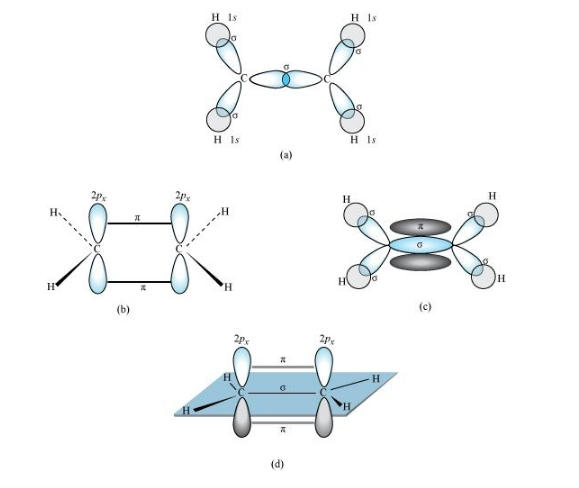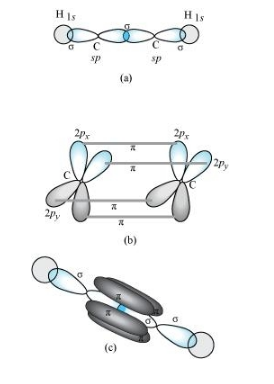# Draw diagrams showing the formation of a double bond and a triple bond between carbon atoms

Question:

Draw diagrams showing the formation of a double bond and a triple bond between carbon atoms in C2H4 and C2H2 molecules.

Solution:

C2H:

The electronic configuration of C-atom in the excited state is:

${ }_{6} \mathrm{C}=1 s^{2} 2 s^{\prime} 2 p_{x}^{\prime} 2 p_{y}^{\prime} 2 p_{z}^{\prime}$

In the formation of an ethane molecule (C2H4), one sp2 hybrid orbital of carbon overlaps a sp2 hybridized orbital of another carbon atom, thereby forming a C-C sigma bond.

The remaining two sp2 orbitals of each carbon atom form a sp2-s sigma bond with two hydrogen atoms. The unhybridized orbital of one carbon atom undergoes sidewise overlap with the orbital of a similar kind present on another carbon atom to form a weak π-bond.C2H2 :

In the formation of C2H2 molecule, each C–atom is sp hybridized with two 2p-orbitals in an unhybridized state.

One sp orbital of each carbon atom overlaps with the other along the internuclear axis forming a C–C sigma bond. The second sp orbital of each C–atom overlaps a half-filled 1s-orbital to form a σ bond.

The two unhybridized 2p-orbitals of the first carbon undergo sidewise overlap with the 2p orbital of another carbon atom, thereby forming two pi (π) bonds between carbon atoms. Hence, the triple bond between two carbon atoms is made up of one sigma and two π-bonds.